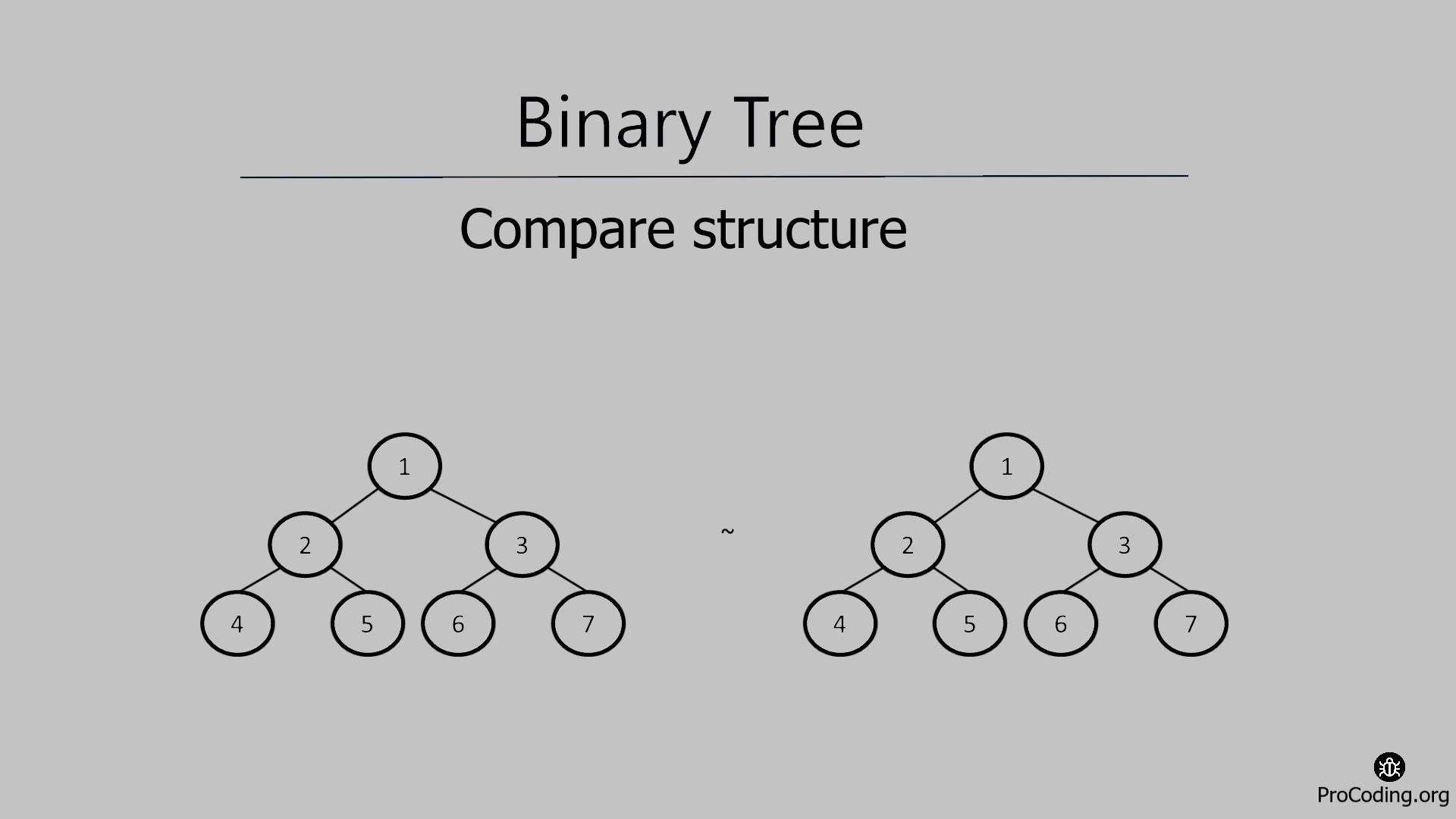# Compare structure of two binary trees

Abhishek Kumar Category: Data StructuresTags: datastructures tree binary-tree

Write a program to compare the structure of two binary trees without data and if they are identical then return true otherwise false by checking each node in the given binary trees.In this problem, we will try to compare the structure of two binary trees (structure only, not the data) and if the structure of both the binary trees is the same then return true otherwise false.

So we have to compare just the structure of both the given trees irrespective of the data and return the result accordingly to check if the trees are identical or not.

##### Example -
```  Input:
1                 5
/   \             /   \
2     3           4     6
/     / \         /     / \
4     6   7       3     2   1

Output: True

Input:
1                 5
/   \             /   \
2     3           4     6
/     / \               / \
4     6   7             2   1

Output: False
```

### Solution

To check if two trees are identical or having the same structure, we need to traverse both trees simultaneously, and while traversing we need to compare the presence of child/children of the trees.

###### Algorithm
Compare_Structure(root1, root2)
``````1. if root1 is null AND root2 is null then
2.    return true
3. if (root1 is not null AND root2 is not null) then
4.   return Compare_Structure(root1.left, root1.right) AND
return Compare_Structure(root2.left, root2.right)
5. return false``````
``````class Node:
def __init__(self, data):
self.left = None
self.data = data
self.right = None

def compare_structure(root1, root2):
if root1 is None and root2 is None:
return True

if (root1 and root2):
return compare_structure(root1.left, root1.right) and compare_structure(root2.left, root2.right)

return False

root1 = Node(1)
root1.left = Node(2)
root1.right = Node(3)
root1.left.left = Node(4)
root1.left.right = Node(5)
root1.right.left = Node(13)
root1.right.right = Node(12)

root2 = Node(1)
root2.left = Node(2)
root2.right = Node(3)
root2.left.left = Node(4)
root2.left.right = Node(5)
root2.right.left = Node(6)
root2.right.right = Node(7)

print(compare_structure(root1, root2))``````
``````class Node {
constructor(data) {
this.left = null;
this.data = data;
this.right = null;
}
}

function compareStructure(root1, root2) {
if (root1 == null && root2 == null) return true;

if (root1 && root2) {
return (
compareStructure(root1.left, root1.right) &&
compareStructure(root2.left, root2.right)
);
}

return false;
}

const root1 = new Node(1);
root1.left = new Node(2);
root1.right = new Node(3);
root1.left.left = new Node(4);
root1.left.right = new Node(5);
root1.right.left = new Node(6);
root1.right.right = new Node(7);

const root2 = new Node(1);
root2.left = new Node(2);
root2.right = new Node(3);
root2.left.left = new Node(4);
root2.left.right = new Node(5);
root2.right.left = new Node(6);
root2.right.right = new Node(7);

console.log(compareStructure(root1, root2));``````

#### Output

Output

`True`

Time complexity: Assuming first binary tree has 'n' nodes and second binary tree has 'm' nodes then node count of the tree having less number of nodes will be considered for time complexity i.e., if n < m then time complexity will be O(n) otherwise it will be O(m).

AUTHOR### Abhishek Kumar

Software engineer | Blogger | Keen learner
Python | Django | JavaScript | React | Next.js | Express.js | C
Passionate about learning and experimenting with new things and open to more opportunities and collaborations.